Function Repository Resource:

# RootSymbol

Recursively take the head of an expression

Contributed by: Ian Ford (Wolfram Research)
 ResourceFunction["RootSymbol"][expr] recursively takes the Head of expr until the Head is Symbol.

## Details and Options

If expr is a symbol, ResourceFunction["RootSymbol"][expr] gives expr.
If expr is any other atomic expression, ResourceFunction["RootSymbol"][expr] is equivalent to Head[expr].
For compound expressions of the form f[][], ResourceFunction["RootSymbol"] gives f.

## Examples

### Basic Examples (3)

The root symbol of a symbol is itself:

 In:=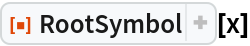Out=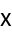The root symbol of an atomic expression is its head:

 In:=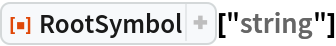Out=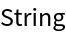Find the root symbol of a compound expression:

 In:=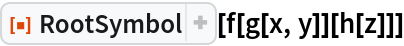Out=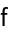## Requirements

Wolfram Language 11.3 (March 2018) or above

## Version History

• 1.0.0 – 07 February 2019